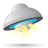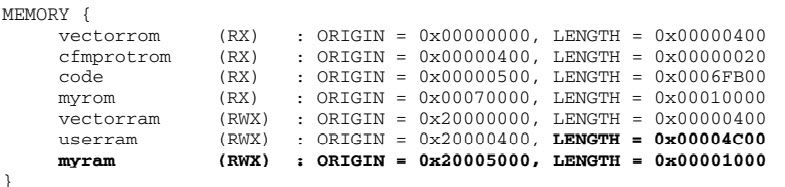## Regarding the End of Line Expected errors for pragma definition in coldfire

cancel
Showing results for
Search instead for
Did you mean:

## Regarding the End of Line Expected errors for pragma definition in coldfire

258 ViewsContributor III

Hi,

I want to relocate a function to a RAM location. I referred AN4329 and used the  Method 1 but I am getting compilation errors as end of line expected for all the three pragama statements. . I did try another method as defined below under Method 2, I am gettign errors for end of line expected along with illegal or unsupported attribute

Please suggest what is the best or any alternative to relocate a function to a RAM location

Method 1

/////defined in .c file

#pragma define_section mySectionInRAM “.myCodeInRAM” far_absolute RX
#pragma section mySectionInRAM begin

void function1(void);

void function1(void)

{

--

}

#pragma section mySectionInRAM end

Method 2

#pragma section RX “.myCodeInRAM” data_mode=far_abs
//#pragma section mySectionInRAM begin

__declspec(section ".myCodeInRAM") void function1(void);

__declspec(section ".myCodeInRAM") void function1(void)

{

}

Also my linker file where I created new section myCodeInRAM

#/*
# * File: flash.dld
# * Purpose: Linker file for M5475EVB and M5485EVB
# *
# * Notes: Creates a Flash bootable image
# */

KEEP_SECTION {.vectortable}

MEMORY
{
boot (RWX) : ORIGIN = 0x00000000 , LENGTH = 0x00200000
text (RWX) : ORIGIN = 0x02001000 , LENGTH = 0x02000000
vector_ram (RWX) : ORIGIN = 0x20000000, LENGTH = 0x00000400 /* Vector table */
data (RWX) : ORIGIN = AFTER(text), LENGTH = 0
myram (RWX) : ORIGIN = AFTER(data), LENGTH = 0
bss (RWX) : ORIGIN = AFTER(myram), LENGTH = 0
}

SECTIONS
{
# Heap and Stack sizes definition
___heap_size = 0x100;
___stack_size = 0x100;

.vector_ram : {} > vector_ram

___MBAR = 0x10000000;
__MBAR = ___MBAR ;

___SDRAM = 0x02000000 ;
___SDRAM_SIZE = (8 * 1024 * 1024);

# ___SDRAM_SIZE = (16 * 1024 * 1024);

___SYS_SRAM = ___MBAR + 0x00010000;
___SYS_SRAM_SIZE = (32 * 1024) ;

___MCDAPI_START = ___SYS_SRAM;
___MCDAPI_SIZE = (12 * 1024);

___CORE_SRAM0 = 0x20000000 ;
___CORE_SRAM0_SIZE = (4 * 1024) ;
__CORE_SRAM0 = ___CORE_SRAM0;

___CORE_SRAM1 = 0x20001000 ;
___CORE_SRAM1_SIZE = (4 * 1024) ;
__CORE_SRAM1 = ___CORE_SRAM1 ;
__CORE_SRAM1_SIZE = ___CORE_SRAM1_SIZE;

___CODE_FLASH = 0x00000000 ;
___CODE_FLASH_SIZE = (32 * 1024 * 1024);
___FLASH = ___CODE_FLASH ;

___BOOT_FLASH = 0x00000000 ;
___BOOT_FLASH_SIZE = (2 * 1024 * 1024);

# ___VECTOR_RAM = ___SDRAM;
___VECTOR_RAM = ADDR(.vector_ram);

# ___SP_AFTER_RESET = ___CORE_SRAM1 + ___CORE_SRAM1_SIZE - 4;

.boot :
{
. = ALIGN(0x4);
_BOOT_BEGIN = .;
_VECTOR_BEGIN = .;
vectors_s.obj(.text)
_VECTOR_END = .;
mcf548x_lo_s.obj(.text)
mcf5xxx_s.obj(.text)
sysinit_c.obj(.text)
. = ALIGN(0x4);
_BOOT_END = .;
} > boot

_VECTOR_SIZE = _VECTOR_END - _VECTOR_BEGIN;

.text : AT(0x00010000)
{
. = ALIGN(0x4);
_TEXT_BEGIN = . ;
#vectors_s.obj(.text)
*(.text)
*(.rodata)
. = ALIGN(0x4);
___DATA_ROM = . ;
_TEXT_END = . ;
} > text

_TEXT_PHYSICAL = 0x00010000;
_TEXT_VIRTUAL = _TEXT_BEGIN;
_TEXT_SIZE = _TEXT_END - _TEXT_BEGIN;

.data : AT(0x00010000 + SIZEOF(.text))
{
. = ALIGN(0x4);
___DATA_RAM = . ;
*(.data)
*(.sdata)
*(.relocate_code)
*(.relocate_const)
*(.relocate_data)
. = ALIGN(0x4);
___DATA_END = . ;
} > data

_DATA_PHYSICAL = 0x00010000 + SIZEOF(text);
_DATA_VIRTUAL = ___DATA_RAM;
_DATA_SIZE = ___DATA_END - ___DATA_RAM;

.my_ram : AT(0x00010000 + SIZEOF(.text) + SIZEOF(data))
{
. = ALIGN (0x4);
___myRAMStart = .;
*(.myCodeInRAM)
___myRAMEnd = .;
. = ALIGN (0x4);
} > myram

_myRAM_PHYSICAL = 0x00010000 + SIZEOF(text) + SIZEOF(data);
_myRAM_VIRTUAL = ___myRAMStart;
_myRAM_SIZE = ___myRAMEnd - ___myRAMStart;

.bss :
{
. = ALIGN(0x4);
___BSS_START = . ;
*(.sbss)
*(SCOMMON)
*(.bss)
*(COMMON)
. = ALIGN(0x4);
___BSS_END = . ;
} > bss

___HEAP_START = .;
___HEAP_END = ___HEAP_START + (512*1024);
___SP_END = ___HEAP_END;
___SP_INIT = ___SP_END + (4*1024);
__SP_INIT = ___SP_INIT;

}

4 Replies
247 ViewsNXP TechSupport

Hi

Please try to change the lcf file with memory size.

For example:Mike

240 ViewsContributor III

Hi,

Please suggest on those lines. I wil try to change as per your suggestiosn not sure it will fix end of line unexpected compilation errors

242 ViewsContributor III

Hi Hui,

Thanks for the update but I am getting pragama complication errors as End of line expected using code warrior when using pragma statements to relocate as per the the document mentioned

213 ViewsNXP TechSupport

Hi,

Could you please help to post the error info screen copy?

I will check if there with any suggestion within internal system.

best regards,

Mike